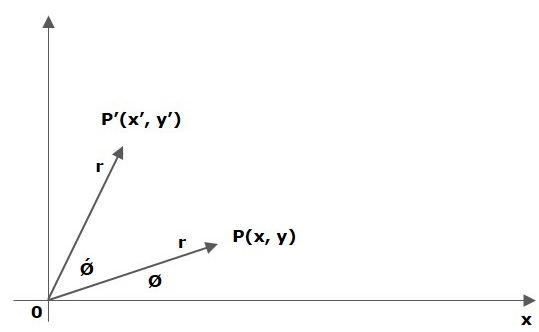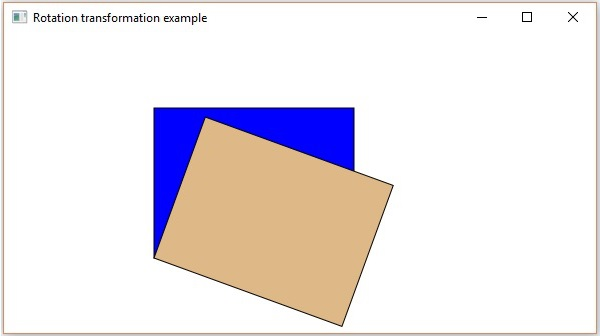# JavaFX - Rotation Transformation

In rotation, we rotate the object at a particular angle θ (theta) from its origin. From the following figure, we can see that the point P(X, Y) is located at angle φ from the horizontal X coordinate with distance r from the origin.## Example

Following is the program which demonstrates the rotation transformation in JavaFX. In here, we are creating 2 rectangular nodes at the same location, with the same dimensions but with different colors (Blurywood and Blue). We are also applying rotation transformation on the rectangle with Blurywood color.

Save this code in a file with the name RotationExample.java.

```import javafx.application.Application;
import javafx.scene.Group;
import javafx.scene.Scene;
import javafx.scene.paint.Color;
import javafx.scene.shape.Rectangle;
import javafx.scene.transform.Rotate;
import javafx.stage.Stage;

public class RotationExample extends Application {
@Override
public void start(Stage stage) {
//Drawing Rectangle1
Rectangle rectangle1 = new Rectangle(150, 75, 200, 150);
rectangle1.setFill(Color.BLUE);
rectangle1.setStroke(Color.BLACK);

//Drawing Rectangle2
Rectangle rectangle2 = new Rectangle(150, 75, 200, 150);

//Setting the color of the rectangle
rectangle2.setFill(Color.BURLYWOOD);

//Setting the stroke color of the rectangle
rectangle2.setStroke(Color.BLACK);

//creating the rotation transformation
Rotate rotate = new Rotate();

//Setting the angle for the rotation
rotate.setAngle(20);

//Setting pivot points for the rotation
rotate.setPivotX(150);
rotate.setPivotY(225);

//Creating a Group object
Group root = new Group(rectangle1, rectangle2);

//Creating a scene object
Scene scene = new Scene(root, 600, 300);

//Setting title to the Stage
stage.setTitle("Rotation transformation example");

stage.setScene(scene);

//Displaying the contents of the stage
stage.show();
}
public static void main(String args[]){
launch(args);
}
}
```

Compile and execute the saved java file from the command prompt using the following commands.

```javac RotationExample.java
java RotationExample
```

On executing, the above program generates a javaFx window as shown below.javafx_transformations.htm